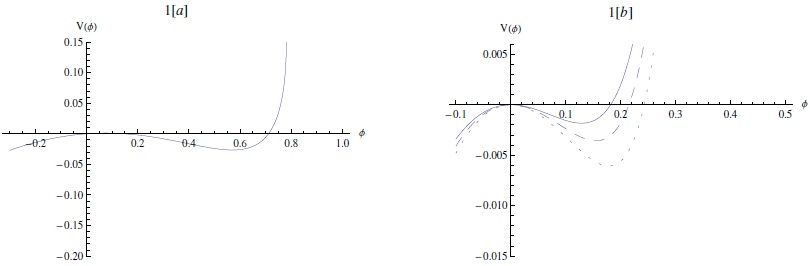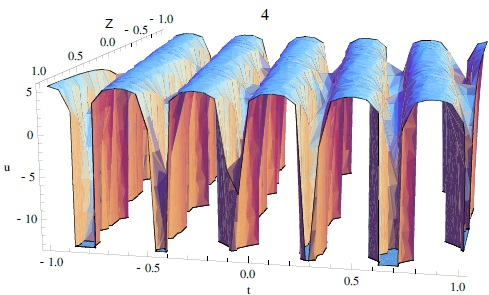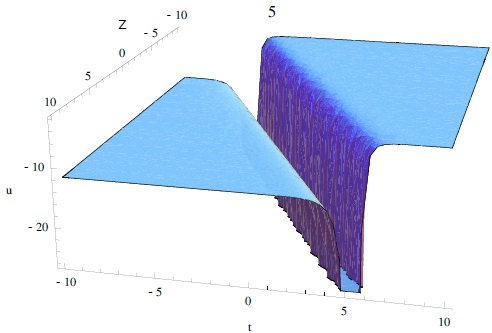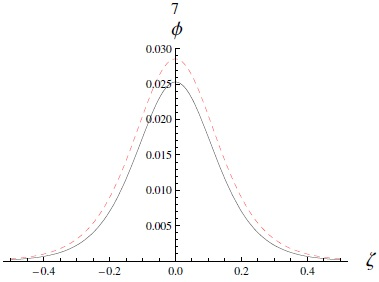## Indicators

•Cited by SciELO
•Access statistics

•Similars in SciELO

## Print version ISSN 0185-1101

### Rev. mex. astron. astrofis vol.54 n.2 México Oct. 2018

Articles

Exact traveling wave solutions of KDV equation for daws in superthermal plasma

1 Department of Mathematics, Faculty of Science, Fayoum University, Fayoum, Egypt.

Abstract

A propagation of fully nonlinear dust-acoustic waves (DAWs) in superthermal plasma is investigated. The plasma is described by the hydrodynamic dust fluid equations with superthermal electrons and ions, where both follow a kappa distribution. The reductive perturbation method is used to analyze small but finite amplitudes nonlinear DAWs. Extended homogeneous balance method is applied to obtain the exact traveling wave solutions for the Korteweg-de Vries (KdV) equation. The solutions are numerically analyzed to study the characteristics of arbitrary and small but finite amplitudes DAWs. This study is important for understanding the nonlinear excitations that may appear in astrophysical plasma objects such as the Jupiter magnetosphere.

Key Words: hydrodynamics; plasmas; waves

Resumen

Se investiga la propagación de ondas acústicas con polvo no lineales (DAWs) en un plasma supratérmico. Se describe el plasma con las ecuaciones hidrodinámicas de un fluido con polvo, incluyendo electrones supratérmicos e iones, ambos con una distribución kappa. Se usa el método reductivo de perturbaciones para analizar DAWs no lineales con amplitudes pequeñas pero finitas. Se aplica el método de balance homogéneo extendido para obtener las soluciones exactas de las ecuaciones de Korteweg-de Vries (KdV) para la propagación de ondas. Se analizan numéricamente las soluciones para estudiar las características de los DAWs con amplitudes arbitrarias y pequeñas. Este estudio es importante para entender las excitaciones no lineales que pueden presentarse en plasmas astrofísicos, como la magnetosfera de Júpiter.

1. INTRODUCTION

Nowadays the κ distribution is the focus in the model of space plasmas (Hasegawa et al. 1985; Sabry et al. 2012; Saini and Kourakis 2008; Saini et al. 2009; Saini and Kourakis 2010). Note that for all velocities, the κ distribution function approaches the Maxwellian distribution (which is considered a special case) for very large κ (κ → ∞). Particles described by κ distribution are found in many kinds of plasma environments like the solar wind, the Earth’s magnetospheric plasma sheet, Jupiter, and Saturn (Abdelsalam 2013; El-Shewy et al. 2011; Hellberg and Mace 2002; Leubner 2004; Sabry et al. 2012).

The inclusion of superthermal particles in a plasma characterizes the high energy region in space, and the presence of superthermal particles causes velocity space diffusion (Hasegawa et al. 1985). Many authors used the kappa distribution to study superthermal particles (ions, positrons, and electrons) (Abdelsalam et al. 2008; Ali et al. 2007; Pakzad 2011). A one-dimensional kappa distribution along a preferred direction in space and a Maxwellian distribution perpendicular to it were the focus of many studies (Hellberg and Mace 2002; Kourakis and Williams 2013). However, few works dealt with superthermal ions (as in Hellberg and Mace 2002; Saini and Kourakis 2008). Kappa distributions are more suitable to study the solar wind (Pierrard and Lemaire 1996), Jupiter and Saturn (Krimigis et al. 1983) or to explain spacecraft data in the Earth’s magnetospheric plasma sheet (Christon et al. 1988) and the velocity filtration effect in the solar corona (Pierrard and Lemaire 1996).

Schippers et al. (2008) analyzed the CAPS/ELS and MIMI/ LEMMS data from the Cassini spacecraft orbiting Saturn over a range of 5.420 Rs, where Rs ≈ 60300 km is the radius of Saturn (Alam et al. 2013). For Saturn’s F-ring the nonlinear structure could be due to solitons for a very short range of plasma parameters and the largest possible solitary waves generated in plasma system with kappa-distribution with a specific velocity distribution are found to be smaller than those found in a Maxwell-Boltzmann plasma (Pakzad 2011; Saini et al. 2009; Shukla and Silin 1992). Recently, DAWs became interesting for nonlinear waves because dust particles are found in interstellar clouds (Abdelsalam 2013; El-Shewy et al. 2011; Leubner 2004; Moslem et al. 2010; Sabry et al. 2012; Shukla and Silin 1992). Depending on the nature of the charge and mass density of the dust grains, it is possible to calculate the phase velocity and the corresponding frequency for any wave as well as for a plasma system.

Here, we consider a simple model discussed in § 2 to study the effective superthermal phenomena for both electrons and ions in astrophysical compact objects and space. First, we derive the Sagdeev potential to study the large amplitude wave structure in § 3. We also derive the KdV equation with its solution for solitary nonlinear waves using a reductive perturbation method as discussed in § 4. Finally an extended homogeneous balance method is used to solve the KdV equation (Abdelsalam et al. 2008; Abdelsalam 2010; Pakzad 2011) in § 5. The numerical analysis is presented in § 6. The conclusion is summarized in § 7.

2. BASIC EQUATIONS

We considered a plasma system containing both superthermal electrons and ions with a kappa distribution, and heavy dust particles positively charged, i.e., our plasma model is composed of dust, superthermal ions and electrons. The nonlinear propagation of the electrostatic DAWs is described by

ndt+xndud=0, (1)

udt+ududx+ϕx=0, (2)

2ϕx2=ne-nd-ni, (3)

where the ions and electrons obey the κ distribution law (Pakzad 2011) as follows:

ne=1-ϕκ-3/2-κ+12, (4)

and

ni=μi1-σϕκ-3/2-κ+12, (5)

where κ is a real parameter measuring the deviation of the potential energy from the Boltzmann distribution. In the limit κ → ∞, the superthermal distribution reduces to the Boltzmann distribution.

In the last equations, nj is the number density (where j = i,d,e), ud is the dust fluid velocity, ϕ is the electrostatic potential, and σ = Te /Ti is the unperturbed electron-to-ion temperature ratio. At equilibrium, we have μd + μi = 1, where μd = nd0 /ne0, μi = ni0/ne0, and nd0 is the unperturbed dust number density (Abdelsalam et al. 2008).

3. LARGE AMPLITUDE OF THE SAGDEEV-LIKE POTENTIAL AND NUMERICAL ANALYSIS

First, we derive a Sagdeev-type pseudo-potential as an energy balance-like equation (Abdelsalam 2010) and then we numerically solve the equation to study the properties of both the Sagdeev-like potential and a solitary pulse.

To study the finite amplitude DASWs and their properties, we assume that the dependent variables in equations (1)-(5) depend on one variable η = xMt, where M is the Mach number (soliton velocity/Csd) and η has been normalized by λD. Thus, we obtain from equations (1) and (2) that nd=μd1-2ϕM2-1. Then, by substituting nd, ne and ni into Poisson equation [equation (3)], and multiplying the left and right sides of the obtained equation by dϕ/dη, we can integrate the equation and apply the boundary conditions, ϕ → 0 and dϕ/dη → 0 at η → ±∞; we get

12dϕdη2+Vϕ=0, (6)

where the Sagdeev potential for our purposes reads

Vϕ=1-1-ϕκ-3/2-κ+32-μiσ1-1-σϕκ-3/2-κ+32+μdM21-1-2ϕM21/2. (7)

Equation (7) can be regarded as an ‘energy integral’ of an oscillating particle of unit mass, with a velocity dϕ/dη and position ϕ in a potential V(ϕ).

If the DASWs exist, then the Sagdeev potential needs to satisfy the following conditions: (a) at ϕ = 0, d2 V/dϕ2 < 0; (b) we can find a critical value (max. or min. value of ϕ) ϕm, at which V(ϕm) = 0; and (c), when V(ϕ) < 0 then we find 0 < ϕ < ϕm. So we can obtain the maximum and minimum values of the Mach number M for which solitons exist. Using the condition (a), the lower limit of Mach number M is 2κ-32κ-1μd1-σμi. The maximum number of M can be obtained by the condition V(ϕc) ≥ 0, where ϕc = M 2/2 is the minimum value of ϕ for which the ion density is real. Thus, we have

1-M22κ-3-κ+32-1-μiσ1-σM22κ-3-κ+32-1>0.

The latter inequality provides the maximum M, which turns out to depend on the parameters μi and σ. Therefore, the following inequality can help us to calculate the Mach number range

2κ-32κ-1μd1-σμi<M<1-M22κ-3-κ+32-1-μiσ1-σM22κ-3-κ+32-1. (8)

We obtain the range of Mach number as 0.45 < M < 1.2 for the values of μ i = 0.25 and σ = 0.9. The existence of supersonic and subsonic solitons is noted. By increasing the ratio μi, to μi = 0.4, the Mach number range reads 0.45 < M < 0.99, which shows that the increase of μi leads to an expansion of subsonic solitons.

In Figures 1 and 2, the Sagdeev potential in equation (8) has been numerically analyzed. Note that the profile of the potential depends on α and M. Figure 1 shows the effect of the ion-to-electron number density ratio μi on the compressive solitary pulse for subsonic and supersonic waves. An increase of μi (which means a decrease of μd) makes the potential deeper and wider. However, a supersonic soliton has deeper amplitude and wider depth than a subsonic soliton.Fig. 1 The Sagdeev potential V(ϕ) [represented by equation (7)] against the potential ϕ. (a) supersonic solitary pulse for M = 1.1. (b) subsonic solitary pulse for M = 0.8. The effect of varying μi = 0.2 (solid curve), μi = 0.3 (dashed curve), and μi = 0.4 (dotted curve), where σ = 0.9 is shown. The color figure can be viewed online.Fig. 2 The Sagdeev potential V(ϕ) [represented by equation (7)] against the potential ϕ. (a) supersonic solitary pulse for M = 1.1 (solid curve), M = 1.15 (dashed curve), and M = 1.2 (dotted curve). (b) subsonic solitary pulse for M = 0.8 (solid curve), M = 0.85 (dashed curve), and M = 0.9 (dotted curve). Here, μi = 0.3 and σ = 0.95. The color figure can be viewed online.

Figure 2 shows that increasing the Mach number (M) leads to the expansion of the permitted region of the potential values associated with the localized excitations, which implies that faster pulse excitations will be large and narrow. The energy in equation (6) has been numerically solved for many parameters. The profiles of the potential pulse are drawn in Figure 3. It is found that shorter pulses are wider than longer pulses and that increasing M causes an increase in the amplitude and a decrease in the width.Fig. 3 The potential ϕ [the solution of equation (6)] is depicted against ζ. (a) supersonic solitary pulse for M = 1.1 (solid curve) and M = 1.25 (dashed curve). (b) subsonic solitary pulse for M = 0.8 (solid curve) and M = 0.9 (dashed curve). Here μi = 0.2 and σ = 0.8. The color figure can be viewed online.

4. KORTEWEG-DE VRIES EQUATION AND SOLUTION FOR NUMERICAL ANALYSIS

In this section, we study solitary waves with small and finite amplitude deriving the Korteweg-de Vries (KdV) equation using a nonlinear perturbation method to study dust-acoustic-solitary-waves (DASWs) (Abdelsalam 2010).

For the reductive perturbation method, we should use the stretched space-time coordinates ξ = ε1/2(xϑt) and τ = ε3/2t (Washimi and Tanituri 1966), where ϑ is the phase speed and ε is the smallness parameter measuring the weakness of the dispersion (0<ε<1). The dependent variables are expanded as

Ψ=Ψ(0)+n=1ϵnΨ(n), (9)

where n = 0,1,2,3,. . .,

Ψ=ne,nd,ni,ud,ϕT, (10)

and

Ψ(0)=1,μd,μi,0,0T. (11)

Using the last stretching and expansions into equations (1)-(5), we isolate distinct orders in ϵ and derive the corresponding variable contributions. The lowest-order equations in ϵ read

ne(1)=2κ-32κ-1ϕ(1),    ni(1)=μiσ2κ-32κ-1ϕ(1),    nd(1)=μd/ϑ2ϕ(1),   ud(1)=1/ϑϕ(1),

and

ϑ=2κ-32κ-1μd1-σμi .

Note that the minimum Mach number is identically ϑ.

The next order in ϵ yields the values for ne(2), ni(2), ud(d), and nd(2). However, by solving the last equations system, we obtain the KdV equation (Malfliet 1992)

ϕ(1)τ+Aϕ(1)ϕ(1)ξ+B3ϕ(1)ξ3=0, (12)

where A is the nonlinear coefficient and B is the dispersion coefficient, respectively,

A=32ϑ+μiϑ3σ24κ2-12μd2κ-32-ϑ34κ2-12μd2κ-32,    B=ϑ32μd . (13)

5. EXTENDED HOMOGENEOUS BALANCE METHOD TO SOLVE THE KDV EQUATION

The extended homogeneous balance (HB) method is applied to obtain many solutions for the KdV equation (Abdelsalam 2017; Abdel-Rady et al. 2010; El-Wakil et al. 2007; Wang 1995; Wang et al. 1995). Consider the KdV equation [equation (12)] as:

uτ+αuuξ+βuξξξ=0 (14)

where α stands for A and β for B.

Applying the transformation u(ξ,τ) = U(η),η = ξλτ to equation (14), we find that U satisfies the following ordinary differential equation

-λU'+αUU'+βU'''=0. (15)

Integrating (15) with respect to η once, we get

-λU+α2U2+βU''=0. (16)

Now, balancing U 2 with U′′ gives the magnitude, |m| = 2. Hence, the solution should be of the form

U=a0+b0+a1ω+b11+ω-1+a2ω2+b21+ω-2, (17)

where the Riccati equation is, ω′ = k + M ω + P ω2; here, ω is the angular frequency, ω′ = /d/ξ, k is the wave number, and M and P are the coefficient parameters; k and P cannot be zero (0).

Substituting the last equation equation (17) in equation (16), we get a polynomial equation ω. Now, equating the coefficient of ωi (i = 0, 1, 2, ...) to zero, we get a system of algebraic equations and by solving this system using package MATHEMATICA (Abdelsalam 2017), we obtain:

the first set:

α0;   a1=-12MPβα;   a2=-12P2βα;   b1=0;   b2=0;k=-2βM2-αa04Pβ;   λ=βM2+8kPβ+αa0; (18)

the second set:

α0;   a0=42kPβ-5P2βα;   a1=-24P2βα;   a2=-12P2βα;   b1=0;   b2=-12βk2-2Pβk+P2βα;   M=2P;  λ=4βP2+8kβP+αa0; (19)

Four cases regarding the Riccati equation were previously published by Abdelsalam (2017). As full details of these four cases are already published, here they are not discussed. Case I is considered when P = 1 and M = 0. The Riccati equation gives two solutions for k < 0 and k > 0 separately. Case II is considered for balancing between ω′ and ω 2. An arbitrary constant, r = 1, is used for Case III. Finally, balancing ω′ with ω2 leads to |m| = 1, and Case IV is introduced. We will use the case numbers in this work to explain our current study. At this stage, we encourage the readers to consult Abdelsalam (2017).

For the first set of equations (18), if M = 0, P = 1 the obtained solutions will satisfy Case I of the solutions for the Riccati equation (Abdelsalam 2017). For k > 0, the solutions of the KdV equation of the type of equation (14), will be

u1ξ,t=a0-12kβtan2kηα, (20)

u2ξ,t=a0-12kβcot2kηα. (21)

For k < 0,

u3ξ,t=a0+12kβtanh2-kηα, (22)

u4ξ,t=a0-12kβcoth2kηα, (23)

For k = 0,

u5ξ,t=a0-12βαη2, (24)

Now with the compatibility condition, we obtain the solutions satisfying Cases II, III, and IV,

Pk=M2-p124. (25)

Therefore, substituting for P and k, from (18) into (25), and solving for p1, we find that

p1=3βM2+αa0β . (26)

Hence, for Case II, we get the following solutions:

u6ξ,t=a0-3βp12M+2tanhηp12α+6Mβp1M+2tanhηp1α, (27)

and

u7ξ,t=a0-3βM-2cothηp12p12α+6MβM+2cothηp1p1α, (28)

For Case III, the solution is

u8ξ,t=a0+3βαr+coshη2coshη2r+coshηM2+M2-1r2-sinhηsinhη+2r2-1+1, (29)

with the condition that p1 = 1. Finally, for Case IV,

u9ξ,t=a0-3βM+cothη+cschη2α-6MβM+cothη+cschηα, (39)

with the condition that p1 = 1, and

u10ξ,t=3βM2-4coth2η+αa0α, (31)

with the condition that p1 = 2.

For the second set we are left only with solutions satisfying Cases II, III, and IV. Since the main criteria for these cases to be applicable is the compatibility condition,

Pk=M2-p124, (32)

from (19), it is found that

p1=-3βM2-αa02β. (33)

Therefore, solutions to equations of the type of equation (14) will be

u11ξ,t=4βα-3k-P2P2(P-p1P+tanhηp1)2+2k-5PP+6p1P+tanhηp1P-3p12P+tanhηp12, (34)

and

u12ξ,t=4βα-3k-P2P2P-P+cothηp1)p12+2k-5PP+6P+cothηp1p1P-3P+cothηp12p12, (35)

For Case III,

u13ξ,t=βα-48k-P2P2r+coshη2sinhη+r2-12-32P+sinhη+r2-1r+coshη2+42k-5PP+12P2P+sinhη+r2-1r+coshη, (36)

where p1 = 1.

Finally for case IV,

u14ξ,t=βα-48k-P2P24P+cothη+cschη2+42k-5PP-122P+cothη+cschηP-32P+cothη+cschη2, (37)

with p1 = 1 and

u15ξ,t=-4βα3coth4η-2k-PP coth2η+3k-P2P2tanh2η, (38)

with the condition that p1 = 2.

To investigate the nonlinear properties of solitary waves [as represented by equation (22)], for k = −ϑ/4β we express the solution equation (14) in the following form

ϕ(1)=ϕ0sech2ξ, (39)

where the amplitude is ϕ0 = 3u0/A and the width is ∆ = (4B/u0)1/2 for the solitary wave profiles caused by the balance between nonlinearity and dispersion. As the standard result for KdV we see that ϕ02 = 12B/A = constant (for given ϑ) (Killian 2006).

6. NUMERICAL ANALYSIS

The extended HB is applied to give the traveling wave solutions for the KdV equation (Abdelsalam 2017; El-Wakil et al. 2007; Wang 1995). The obtained solutions cover many types of periodical, rational, singular and solitary wave solutions. As an example, the solution of equation (20) is a sinusoidal-type periodical solution shown in Figure 4. The solutions for equation (24) are of rational-type and the solution of equation (22) is a kink-type solitary wave solution. Note here that equations (23) and (31) are explosive/blowup solutions as depicted in Figure 5. The solution of equation (6) represents a soliton wave with amplitude ϕ0.Fig. 4 3D plots of the solution of equation (20) with A = 0.5 and B = 2.9. The color figure can be viewed online.Fig. 5 3D plots of the solution equation (30) with A = 0.5, B = 2.9 and M = 0.9. The color figure can be viewed online.

Note that increasing the electron-to-ion temperature ratio σ = Te/Ti as well as the parameter κ will decrease the amplitude (as in Figure 6), while we can see in Figure 7 the effect of increasing the ion-to-electron number density ratio μi (which means a decrease of μd) which will make the soliton taller and wider. We can now compare this analytical solution to the numerical solution (obtained by numerical integration) of equation (6), in Figure 8. Note that the difference between the approximate and exact solutions increases with increasing nonlinearity, thus enhancing the amplitudes of pulses.Fig. 6 The variation of the solitary pulse amplitude ϕ0 [equation (39)] against κ and σ for fixed values of μi = 0.22, and ϑ = 0.1. The color figure can be viewed online.Fig. 7 The solitary potential excitation [as given by equation (39)] for varying the value of phase speed μi = 0.21 (solid curve) and μi = 0.3 (dashed curve) with κ = 3, σ = 0.9, and ϑ = 0.1. The color figure can be viewed online.Fig. 8 The potential ϕ [exact/numerical integration of equation (7)] and the approximate potential [represented by equation (39)] are depicted against ζ. The solid and dashed curves represent the exact and approximate solutions, respectively. Here μi = 0.2, σ = 0.9, κ = 3, M = 1.1, and ϑ = 0.1. The color figure can be viewed online.

Overall, in this paper the nonlinear dust-acoustic solitary wave propagation is studied in a superthermal ionelectron-dust plasma. The dust is described by the hydrodynamic equations with superthermal electrons and ions. The Sagdeev equation is derived. It is shown that for low values of μi we can observe both supersonic and subsonic DAWs. While increasing μi leads to an expansion of subsonic solitons only, we discussed the reliance of the potential pulse excitation characteristics and of the pseudo-potential profiles on μi and the Mach number. The effects of the physical parameters have been studied on the localized DAWs structures/excitations and the propagating nonlinear structures. The extended homogeneous balance method extracts a wide range of solution structures using the techniques for solving partial differential equation (PDEs); the method is applied to the KdV equation. Many traveling wave solutions are formally derived for these equations, some of them new and interesting (Abdelsalam et al. 2008; Abdelsalam 2010, 2017; El-Wakil et al. 2007; Pakzad 2011; Wang et al. 1995). These solutions include many types of wave solutions.

7. CONCLUSION

In the previous investigations, it was confirmed that theoretically dust particles with positive charges are present in the outer magnetosphere of Jupiter and Saturn (Horanyi et al. 2004; Scarf 1969); the same speed for ions and electrons was considered to study the solitons and shock waves (Saleem et al. 2012). However, the existence of rogue waves was also found when the solar wind interacts with Jupiter’s magnetosphere (Tolba et al. 2015, 2017; Abdelghany et al. 2016) in the presence of positively charged dust particles (Horanyi et al. 2004; Saleem et al. 2012). Thus, nonlinear waves are also present in Jupiter’s magnetosphere and due to the presence of ions and electrons, the streaming dust acoustic wave speed effect does not contribute to the dust dynamics (Horanyi et al. 2004; Scarf 1969; Saleem et al. 2012; Tolba et al. 2015, 2017; Abdelghany et al. 2016). Thus in this work, we consider positively charged dust particles in our superthermal plasma model and study both large and small amplitude nonlinear wave propagation using the reductive perturbation method.

This work is mainly relevant to nonlinear DASWs in superthermal electrons and ions following κ distribution in an unmagnetized collisionless plasma. The dissipation of solitary waves (solution of the KdV equation) in the presence of positively charged dust particles is also studied numerically with the extended homogeneous balance method used to solve the KdV equation. Because of the large range of σ(= Te /Ti), the Landau damping effect on dissipation is negligible, but the damping of solitary waves is mainly caused by the ion-dust collision and the kinematic viscosity (Nakamura and Sarma 2001). Thus if we consider a viscosity term in the model the nonlinear structure for shock waves can be studied(Lu et al. 2006; Nakamura et al. 1999; Pakzad 2011). Some studies considered positrons in the plasma model as super-dense electron-positron-ion plasmas which also can be found in some astrophysical environments (Abdelsalam et al. 2008; Ali et al. 2007; Pakzad 2011).

Acknowledgements

The authors would like to express their deepest gratitude to the reviewer for his constructive comments, which significantly contributed to improve the manuscript. MSZ wants to thank Bangladesh University of Textiles, Dhaka, Bangladesh for this collaboration work.

References

Abdelghany, A. M., Abd El-Razek, H. N., Moslem, W. M., & El-Labany, S. K. 2016, PhPl, 23, 2121 [ Links ]

Abdel-Rady, A. S., Osman, E. S., & Khalfallah, M. 2010, CNSNS, 15, 264 [ Links ]

Abdelsalam, U. M., Moslem, W. M., & Shukla, P. K. 2008, PhLA, 372, 4057 [ Links ]

Abdelsalam, U. M. 2010, PhyB, 18, 3914 [ Links ]

Abdelsalam, U. M. 2013, JPlPh, 79, 287 [ Links ]

Abdelsalam, U. M. 2017, Journal of Ocean Engineering and Science, 2, 28 [ Links ]

Alam, M. S., Masudb, M. M., & Mamun, A. A. 2013 ChPhB, 22, 5202 [ Links ]

Ali, S., Moslem, W. M., Shukla, P. K., & Schlickeiser, R. 2007, PhPl, 14, 2307 [ Links ]

Christon, S. P., et al. 1988, JGR, 93, 2562 [ Links ]

El-Wakil, S. A., Abulwafa, E. M., Elhanbaly, A., & Abdou, M. A. 2007, CSF, 33, 1512 [ Links ]

El-Shewy, E. K., El-Maaty, M. I. A., Abdelwahed, H. G., & Elmessary, M. A. 2011, Ap&SS, 322, 179 [ Links ]

Hasegawa, A., Mima, K., & Duong-van, M. 1985, PhRvL, 54, 2608 [ Links ]

Hellberg, M. A. & Mace, R. L. 2002, PhPl, 9, 1495 [ Links ]

Horanyi, M., et al. 2004, RvGeo, 42, 4002 [ Links ]

Killian, T. C. 2006, Natur 441, 298 [ Links ]

Kourakis, I. & Williams, G. 2013, Modelling of multidimensional electrostatic excitations in electronpositron-ion plasmas in the presence of kappa-distributed superthermal particles; AGU Fall Meeting Abstracts, American Geophysical Union, USA [ Links ]

Krimigis, S. M., et al. 1983, JGR, 88, 8871 [ Links ]

Leubner, M. P. 2004, PhPl, 11, 1308 [ Links ]

Lu, D., Hong, B., & Tian, L. 2006, International Journal of Nonlinear Science, 2, 3 [ Links ]

Malfliet, W. 1992, AmJPh, 60, 650 [ Links ]

Moslem, W. M., Abdelsalam, U. M., Sabry, R., & Shukla, P. K. 2010, NJPh, 12, 3010 [ Links ]

Nakamura, Y., Bailung, H., & Shukla, P. K. 1999, PhRvL, 83, 1602 [ Links ]

Nakamura, Y. & Sarma, A. 2001, PhPl, 8, 3921 [ Links ]

Pierrard, V. & Lemaire, J. 1996, JGR, 101, 7923 [ Links ]

Sabry, R., Moslem, W. M., & Shukla, P. K. 2012, PPCF, 54, 035010 [ Links ]

Saini, N. S. & Kourakis, I. 2008, PhPl, 15, 3701 [ Links ]

Saini, S. A., Kourakis, I., & Hellberg, M. A. 2009, PhPl, 16, 2903 [ Links ]

Saini, N. S. & Kourakis, I. 2010, PPCF, 52, 7 [ Links ]

Saleem, H., Moslem, W. M., & Shukla, P. K. 2012, JGRA, 117, A8 [ Links ]

Scarf, F. L. 1969, P&SS, 17, 595 [ Links ]

Schippers, P. et al. 2008, JGRA, 113, 7208 [ Links ]

Shukla, P. K. & Silin, V. P. 1992, PhyS, 45, 508 [ Links ]

Tolba, R. E., Moslem, W. M., El-Bedwehy, N. A. & El Labany, S. K. 2015, PhPl, 22, 043707 [ Links ]

Tolba, R. E., et al. 2017, ITPS, 45, 2552 [ Links ]

Wang, M. L. 1995, PhLA, 199, 169 [ Links ]

Wang, M. L., Zhou, Y. & Li, Z. 1996, PhLA, 216, 67 [ Links ]

Washimi, H. & Taniuti, T. 1996, PhRvL, 17, 996 [ Links ]

Received: January 29, 2018; Accepted: June 05, 2018

U. M. Abdelsalam: Department of Mathematics, Faculty of Science, Fayoum University, Fayoum, Egypt (usama.ahmad@rub.de).

William M. S. Zobaer: Department of Physics, Bangladesh University of Textiles, Dhaka 1208, Bangladesh (m.s.zobaer@butex.edu.bd).This is an open-access article distributed under the terms of the Creative Commons Attribution License